### 6.2 The Sin­gle-Par­ti­cle States

As the pre­vi­ous sec­tion noted, the ob­jec­tive is to un­der­stand sys­tems of non­in­ter­act­ing par­ti­cles stuck in a closed, im­pen­e­tra­ble, box. To do so, the key ques­tion is what are the sin­gle-par­ti­cle quan­tum states, or en­ergy eigen­func­tions, for the par­ti­cles. They will be dis­cussed in this sec­tion.

The box will be taken to be rec­tan­gu­lar, with its sides aligned with the co­or­di­nate axes. The lengths of the sides of the box will be in­di­cated by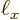,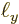, and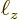re­spec­tively.

The sin­gle-par­ti­cle en­ergy eigen­func­tions for such a box were de­rived in chap­ter 3.5 un­der the guise of a pipe with a rec­tan­gu­lar cross sec­tion. The sin­gle-par­ti­cle en­ergy eigen­func­tions are: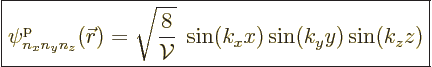(6.2)

Here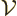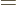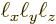is the vol­ume of the box. The “wave num­bers”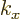,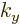, and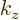take the val­ues: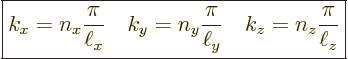(6.3)

where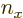,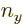, and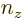are nat­ural num­bers. Each set of three nat­ural num­bers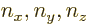gives one sin­gle-par­ti­cle eigen­func­tion. In par­tic­u­lar, the sin­gle-par­ti­cle eigen­func­tion of low­est en­ergy is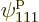, hav­ing1.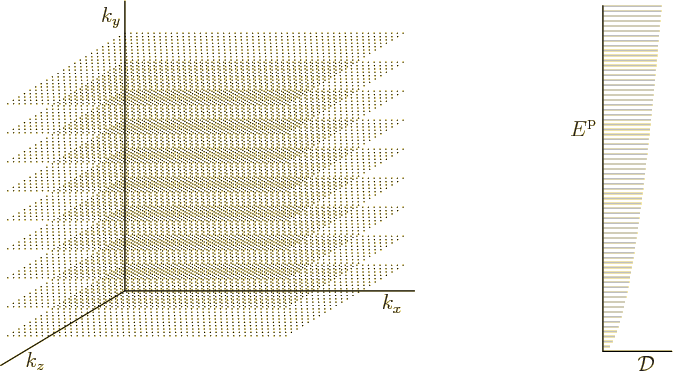How­ever, the pre­cise form of the eigen­func­tions is not re­ally that im­por­tant here. What is im­por­tant is how many there are and what en­ergy they have. That in­for­ma­tion can be sum­ma­rized by plot­ting the al­lowed wave num­bers in a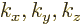axis sys­tem. Such a plot is shown in the left half of fig­ure 6.1.

Each point in this wave num­ber space cor­re­sponds to one spa­tial sin­gle-par­ti­cle state. The co­or­di­nates,, andgive the wave num­bers in the three spa­tial di­rec­tions. In ad­di­tion, the dis­tance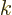from the ori­gin in­di­cates the sin­gle-par­ti­cle en­ergy. More pre­cisely, the sin­gle par­ti­cle en­ergy is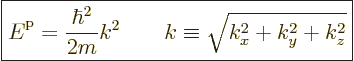(6.4)

The en­ergy is there­fore just a con­stant times the square of this dis­tance. (The above ex­pres­sion for the en­ergy can be ver­i­fied by ap­ply­ing the ki­netic en­ergy op­er­a­tor on the given sin­gle-par­ti­cle wave func­tion.)

One more point must be made. The sin­gle-par­ti­cle en­ergy eigen­func­tions de­scribed above are spa­tial states. Par­ti­cles with nonzero spin, which in­cludes all fermi­ons, can ad­di­tion­ally have dif­fer­ent spin in what­ever is cho­sen to be the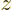-​di­rec­tion. In par­tic­u­lar, for fermi­ons with spin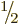, in­clud­ing elec­trons, there is a spin-up” and a “spin-down ver­sion of each spa­tial en­ergy eigen­func­tion: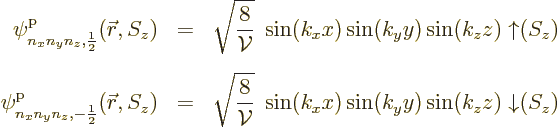That means that each point in the wave num­ber space fig­ure 6.1 stands for two sin­gle-par­ti­cle states, not just one.

In gen­eral, if the par­ti­cles have spin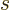, each point in wave num­ber space cor­re­sponds to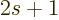dif­fer­ent sin­gle-par­ti­cle states. How­ever, pho­tons are an ex­cep­tion to this rule. Pho­tons have spin1 but each spa­tial state cor­re­sponds to only 2 sin­gle-par­ti­cle states, not 3. (That is re­lated to the fact that the spin an­gu­lar mo­men­tum of a pho­ton in the di­rec­tion of mo­tion can only be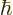or, not 0. And that is in turn re­lated to the fact that the elec­tro­mag­netic field can­not have a com­po­nent in the di­rec­tion of mo­tion. If you are cu­ri­ous, see ad­den­dum {A.21.6} for more.)

Key Points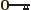Each sin­gle par­ti­cle state is char­ac­ter­ized by a set of three wave num­bers,, and.Each point in the wave num­ber space fig­ure 6.1 cor­re­sponds to one spe­cific spa­tial sin­gle-par­ti­cle state.The dis­tance of the point from the ori­gin is a mea­sure of the en­ergy of the sin­gle-par­ti­cle state.In the pres­ence of nonzero par­ti­cle spin, each point in wave num­ber space cor­re­sponds tosep­a­rate sin­gle-par­ti­cle states that dif­fer in the spin in the cho­sen-​di­rec­tion. For pho­tons, make that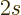in­stead of.